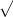math question 19

The number of mirrors that will be produced at a given price can be predicted by the formula

s22x

,

where
s is the supply (in thousands) and
x is the price (in dollars). The demand
d (in thousands) for mirrors can be predicted by the formula

d312 âˆ’ 5x2

.

Find the equilibrium priceâ€”that is, the price at which supply will equal demand.